Question

# 11. The solubility product constant for aluminum hydroxide (Al(OH)3) is 3.0 x 10”. Use the information...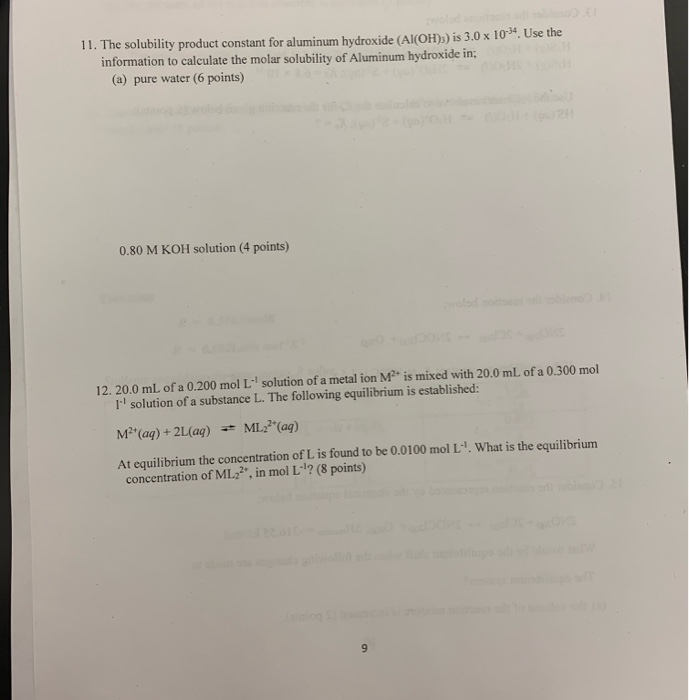11. The solubility product constant for aluminum hydroxide (Al(OH)3) is 3.0 x 10”. Use the information to calculate the molar solubility of Aluminum hydroxide in: (a) pure water (6 points) 0.80 M KOH solution (4 points) 12. 20.0 mL of a 0.200 mol L solution of a metal ion M is mixed with 20.0 mL of a 0.300 mol I'' solution of a substance L. The following equilibrium is established: M (aq) + 2L (aq) + ML(aq) At equilibrium the concentration of L is found to be 0.0100 mol L". What is the equilibrium concentration of ML22*, in mol L '? (8 points)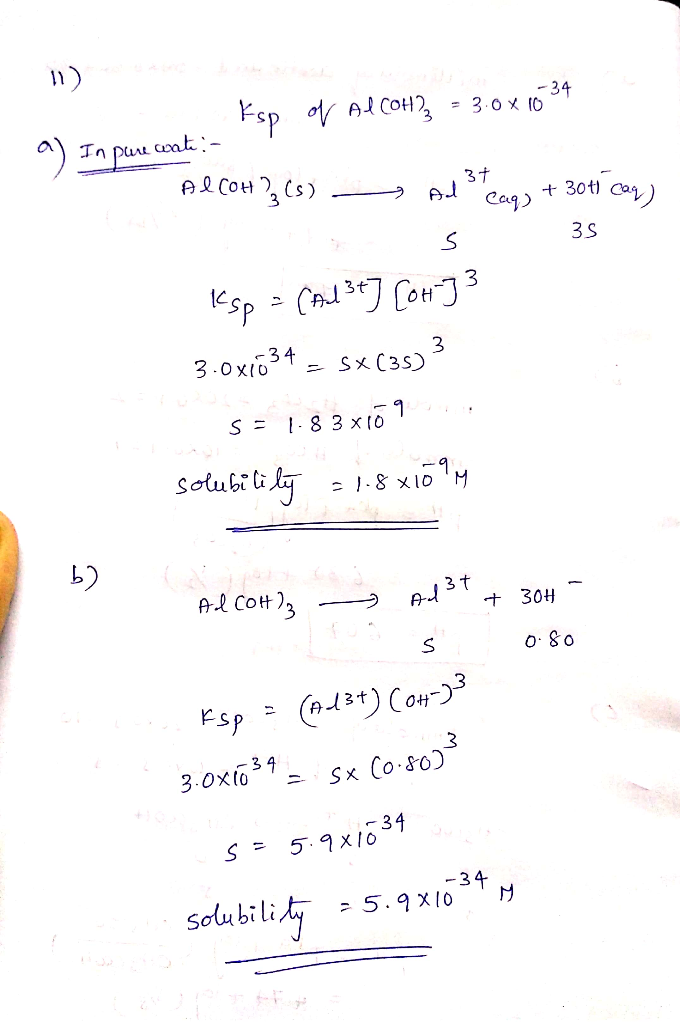#### Earn Coins

Coins can be redeemed for fabulous gifts.

Similar Homework Help Questions
• ### Please give answer with correct sigfigs The solubility product, Ksp, for aluminum hydroxide, Al(OH)3, is 1.9x10-33...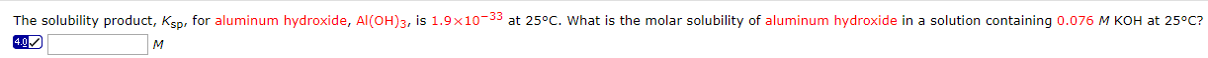Please give answer with correct sigfigs The solubility product, Ksp, for aluminum hydroxide, Al(OH)3, is 1.9x10-33 at 25°C. What is the molar solubility of aluminum hydroxide in a solution containing 0.076 M KOH at 25°C? 4.0 M

• ### 1. (15 pts) Use Ksp value (1E-32) and solubility reaction for aluminum hydroxide, AI(OH)3, found in...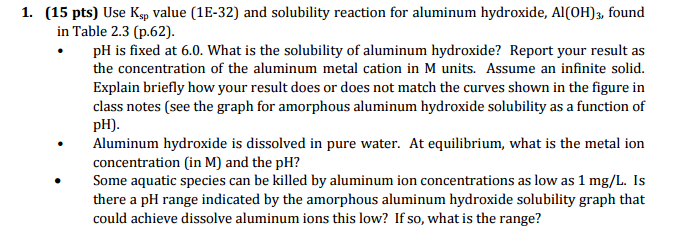1. (15 pts) Use Ksp value (1E-32) and solubility reaction for aluminum hydroxide, AI(OH)3, found in Table 2.3 (p.62). pH is fixed at 6.0. What is the solubility of aluminum hydroxide? Report your result as the concentration of the aluminum metal cation in M units. Assume an infinite solid. Explain briefly how your result does or does not match the curves shown in the figure in class notes (see the graph for amorphous aluminum hydroxide solubility as a function of...

• ### The solubility product, Ksp, for cobalt(III) hydroxide, Co(OH)3, is 1.6X104 at 25°C. What is the molar...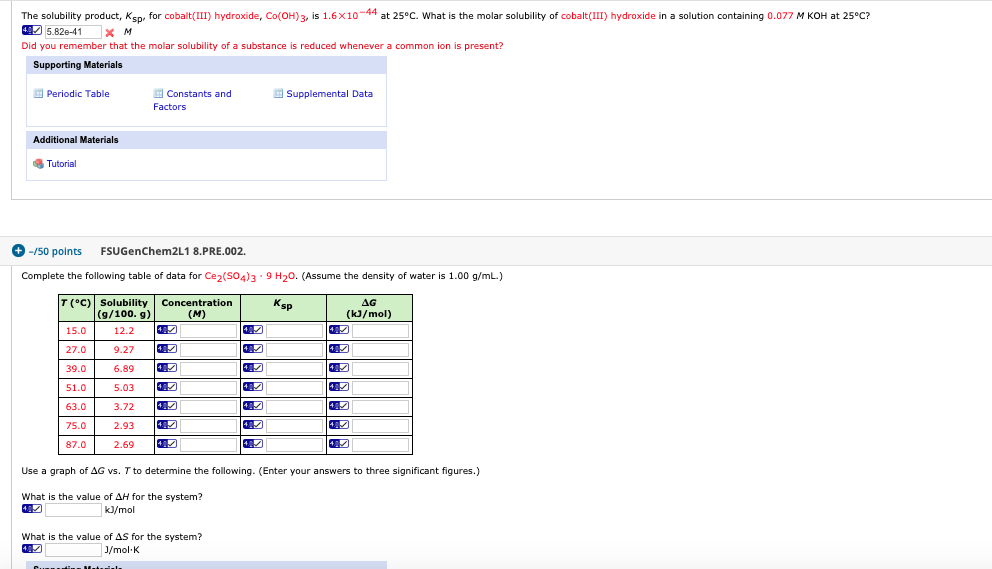The solubility product, Ksp, for cobalt(III) hydroxide, Co(OH)3, is 1.6X104 at 25°C. What is the molar solubility of cobalt(III) hydroxide in a solution containing 0.077 M KOH at 25°C? 125.82e-41 x M Did you remember that the molar solubility of a substance is reduced whenever a common ion is present? Supporting Materials Periodic Table Constants and Factors Supplemental Data Additional Materials Tutorial -/50 points FSUGenChem2L1 8.PRE.002. Complete the following table of data for Ce2(SO4)3 9 H20. (Assume the density of...

• ### Molar Solubility and Solubility Product of Calcium Hydroxide Volume of saturated Ca(OH)2 solution (mL) 25.00...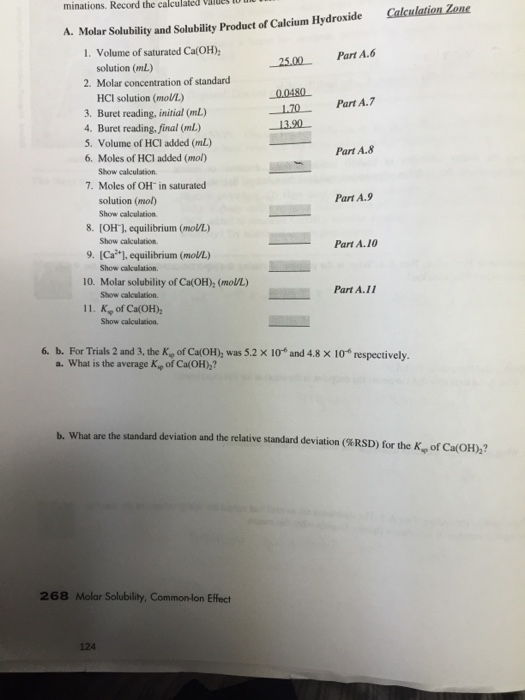Molar Solubility and Solubility Product of Calcium Hydroxide Volume of saturated Ca(OH)2 solution (mL) 25.00 Molar concentration of standard HCI solution (mol/L.) 0.0480 Buret reading, initial (mL) 1.70 Buret reading, final (mL) 13.90 Volume of HCI added (mL) Moles of HCI added (mol) Show calculation Moles of OH^- in saturated solution (mol) Show calculation [OH^-), equilibrium (mol/L) Show calculation. [Ca^2+], equilibrium (mol/L) Show calculation. Molar solubility of Ca(OH)2 (mol/L) Show calculation. Ks rho of Ca(OH)2 Show calculation. For Trials 2...

• ### The solubility product, Ksp, for cobalt(III) hydroxide, Co(OH)3, is 1.6 x 10-44 at 25°C. What is...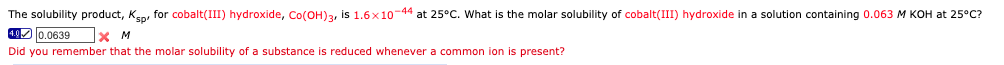The solubility product, Ksp, for cobalt(III) hydroxide, Co(OH)3, is 1.6 x 10-44 at 25°C. What is the molar solubility of cobalt(III) hydroxide in a solution containing 0.063 M KOH at 25°C? 40) 0.0639x M Did you remember that the molar solubility of a substance is reduced whenever a common ion is present?

• ### 17.(10 pts) Calculate the molar solubility of aluminum hydroxide, Al(OH)3 Ksp = 1.9 x 10”, in...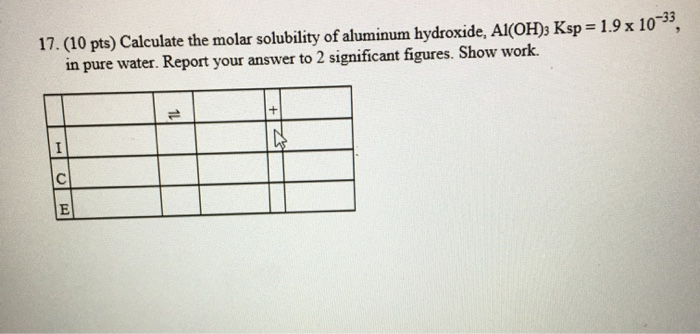17.(10 pts) Calculate the molar solubility of aluminum hydroxide, Al(OH)3 Ksp = 1.9 x 10”, in pure water. Report your answer to 2 significant figures. Show work.

• ### Part A and Part B. Also question #3-post lab. (its circled) A. Molar Solubility and Solubility Product of Calcium Hydroxide Trial I Trial 3 Trial 2 25.0 1. Volume of saturated Ca(OH), solution (m...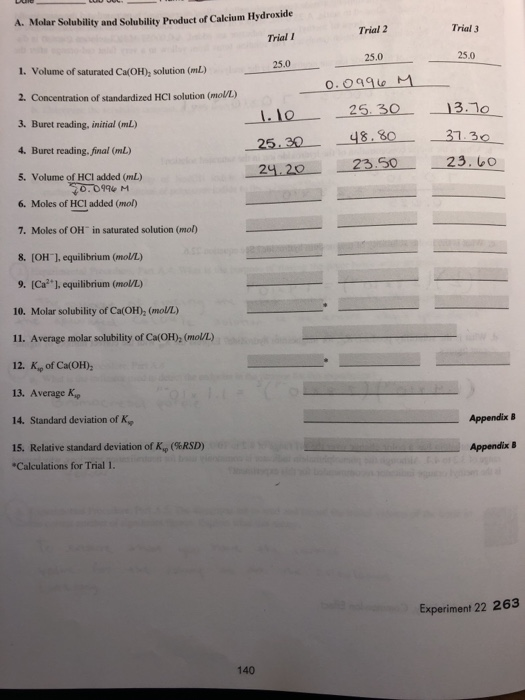Part A and Part B. Also question #3-post lab. (its circled) A. Molar Solubility and Solubility Product of Calcium Hydroxide Trial I Trial 3 Trial 2 25.0 1. Volume of saturated Ca(OH), solution (mL) 2. Concentration of standardized HCl solution (molU/L) 3. Buret reading, initial (mL 4. Buret reading, final (mL) 5. Volume of HCI added (mL) 6. Moles of HCI added (mol) 7. Moles of OH" in saturated solution (mol) 8. (OH1, equilibrium (mol/L) 9. (Ca2 ], equilibrium (mol/L)...

• ### A determination the molar solubility and the K_sp calcium hydroxide was completed according to the experimenta...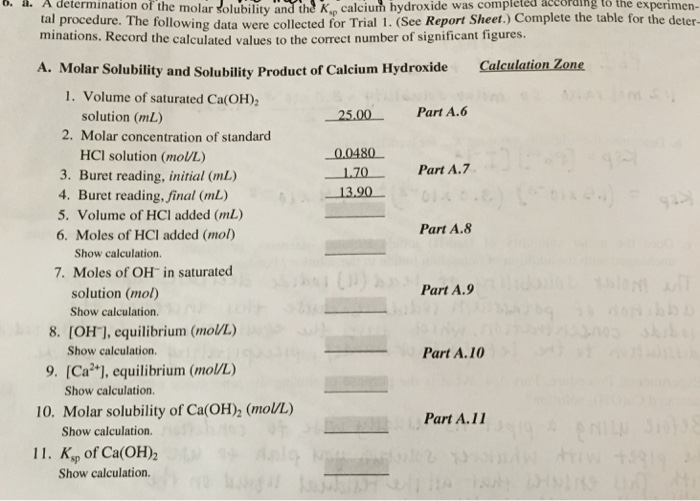A determination the molar solubility and the K_sp calcium hydroxide was completed according to the experimental procedure. The following data were collccicd for Trial 1 (Sec Repon sheet. minations. Record the calculated values to the correct number of significant ligures. A. Molar Solubility and Solubility Product of Calcium Hydroxide 1.Volume of saturated Ca(OH)_2 solution (mL) 2.Molar concentration of standard HCI solution (mol/L) 3.Buret reading, initial (mL) 4.Buret reading, final (mL) 5.Volume of HCI added (mL) 6.Moles of HCI added (mol)...

• ### 1. The Molar Solubility of Ca(10), in Pure Water Temperature of the saturated solution of calcium...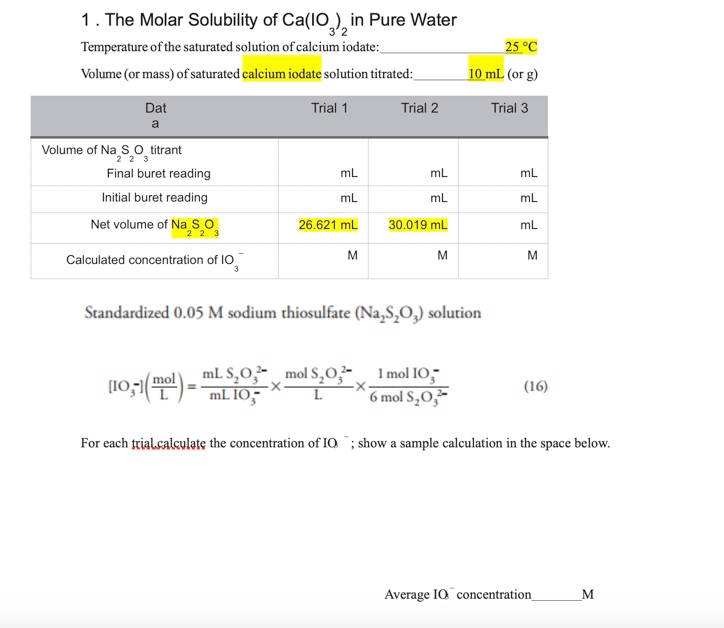1. The Molar Solubility of Ca(10), in Pure Water Temperature of the saturated solution of calcium iodate: Volume (or mass) of saturated calcium iodate solution titrated: 25 °C 10 ml (or g) Dat Trial 1 Trial 2 Trial 3 Volume of Na S o titrant Final buret reading Initial buret reading ml mL mL mL Net volume of Na So 26.621 mL 30.019 mL Calculated concentration of 10 Standardized 0.05 M sodium thiosulfate (Na,8,0,) solution (10,51(E) - TIL 10 mols.0.2...

• ### The solubility product, Ksp, for cobalt(III) hydroxide, Co(OH)3, is 1.6 x 10-44 at 25°C. What is...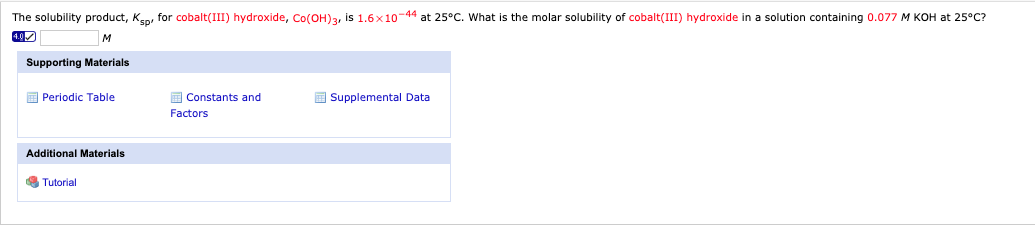The solubility product, Ksp, for cobalt(III) hydroxide, Co(OH)3, is 1.6 x 10-44 at 25°C. What is the molar solubility of cobalt(III) hydroxide in a solution containing 0.077 M KOH at 25°C? Supporting Materials Periodic Table Supplemental Data Constants and Factors Additional Materials Tutorial Complete the following table of data for Cez(SO4)3.9 H20. (Assume the density of water is 1.00 g/ml.) Кsp AG (kJ/mol) T(°C)| Solubility Concentration (g/100.9) (M ) 15.0 12.2 9.27 40 4.9 ) 27.0 40/ 39.0 6.89 40...